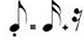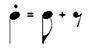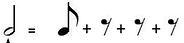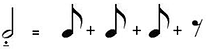# What is Staccato?

The Staccato (or diminution dot) is a dot placed below or above the note. Its function is to divide the value of a figure into sound and silence of the same duration. Observe:

## Example of a StaccatoIn this example, the diminution dot was placed on an eighth note. Notice how it divided the figure into a sixteenth note and a sixteenth rest. The two figures added together add up to an eighth note. Note that the function of this dot is to halve the length of a note, so we are left with a figure in half added to a rest.

Now look at another example, this time on a quarter note:The idea remained the same, the only difference in this example is that the dot was placed above the note. This does not alter the function of the dot at all.

In addition to this type of Staccato, there are also 2 other types, the staccatissimo (or staccato grande) and the half-staccato.

The staccatissimo is shaped like a triangle that points to the head of the note. This dot divides the note into 4 parts of the same length, where the first part is a note and the other 3 parts are silence. See the example below:

## StaccatissimoNotice how the half note, in this case, was divided into 4 eighth notes, where the first was a note and the last 3 were silences.

The half-staccato (or staccato dolce) is represented by a dot and a dash. Its function is to divide the figure’s value also into 4 parts, but here the first 3 figures are sounds and the last one is silence. See the example below:

## Staccato DolceBack to: Sheet music guide